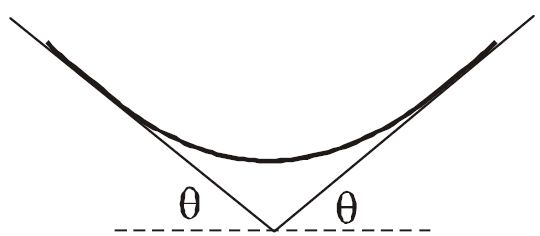# Largest fraction of a rope!A rope rests on two platforms which are both inclined at an angle $\theta$, as shown. The rope has uniform mass density $\lambda$, and its coefficient of friction with the platforms is $\mu=1$. The system has left-right symmetry.

What is the largest possible fraction of the rope that does not touch the platforms?

With $F(\theta) = \sin\theta \cos\theta - \sin^2 \theta$.

×